## Forced Duffing Oscillator

Derivation of the equation:

Duffing equations are used to model certain mechanical or electrical oscillators. One frequently mentioned derivation as a one-mode approximation of the deflections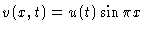of a symmetric beam modelled by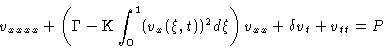with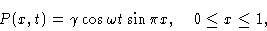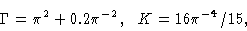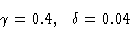is summarized in [R. Seydel: Practical Bifurcation and Stability Analysis. From Equilibrium to Chaos. Second Edition. Springer Interdisciplinary Applied Mathematics 1994.]

It is an instructive exercise to consider the case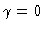(no driving force) with the compressive force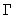as bifurcation parameter. For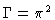there is a pitchfork bifurcation. At this value of Euler's first buckling load the underlying beam starts to bend. Up to symmetry, one stable equilibrium exists for each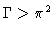. This is satisfied by our parameters.

First-order System:

The harmonic forcing term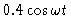on the right-hand side provokes a response of the system (beam, electric current). The harmonic oscillations obey the boundary conditions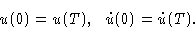Because the period T varies with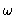, the integration interval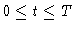must be adapted wheneveris changed. This is inconvenient when nodes of a numerical solution procedure must also be adapted, so we transform the integration interval to unit length, thereby shifting the dependence onto the right-hand side of the differential equation. The normalized time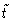satisfies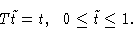The transformation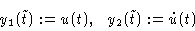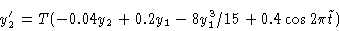with boundary conditions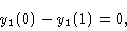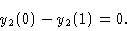Redefining the normalized time by t, we obtain a boundary-value problem of a standard form. With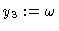, the corresponding extended system is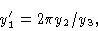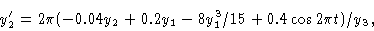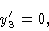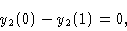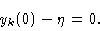Bifurcations:

A main part of the bifurcation diagram is shown in Figures 1, 2. The branching behavior is rich for small values of. As Figure 3 shows, there are many branches, turning points, and bifurcation points for values of the parameter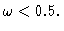The solid curve in this branching diagram represents oscillations, with phase diagrams being symmetric with respect to the origin as in Figure 4. For decreasing, each loop of this main" branch attaches a further wiggle to the oscillation. The wiggles indicate that the small-amplitude oscillation around any of the stable equilibria takes much time to collect enough energy before a transition to the other attracting basin is possible; compare the phase diagram in Figure 4 and the time dependence in Figure 5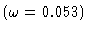. At first glance, the many wiggles might give the impression that such solutions are not harmonic; but they do have period T. Each of the closed branches in Figure 3 (dashed and dotted curves) is attached to the main branch via two pitchfork bifurcations. Accordingly, the phase diagrams of these secondary" branches are asymmetric with respect to the origin; Figure 6 shows one such phase plot. The closed branch in Figure 7 (drawn in Figure 3 in a dashed line) appears to have sharp corners, and one might expect difficulties in tracing this particular branch. The plotted behavior refers only to the dependence y1 (0) versus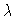; the corresponding graph of y2 (0) behaves more smoothly (Figure 8).

The table includes 20 bifurcation points of the above Duffing equation with accurate values of the amplitude. The above Duffing equation also exhibits subharmonic oscillations; then the period of the system is an integral multiple of the period of the external frequency. Branches of subharmonic solutions bifurcate from the harmonic solutions (not listed in the table). Stability, and period doubling scenarios have been calculated by K. Riedel (Ulm 1996). For example, a period doubling cascade starts at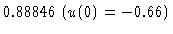.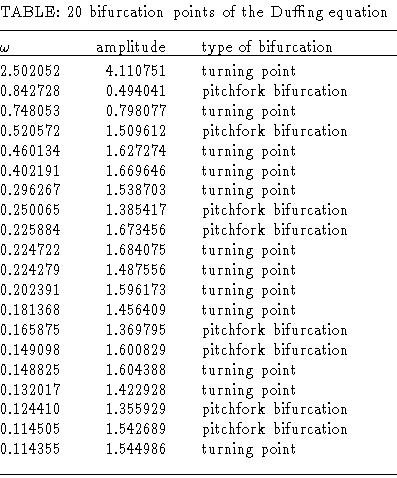Chaotic solutions:

For a range of-values ther exist chaotic attractors. One such attractor is illustrated by the Poincaré map / stroboscopic map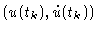,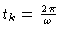, k=1,2,...,8000,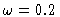,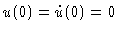in Figure 9.

Figure 1
Bifurcation diagram u(0) versus. Solid curve: branch of symmetric orbits.

Figure 2
Bifurcation diagram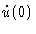versus.

Figure 3
Detail of bifurcation diagram, u(0) versus.

Figure 4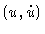phase-plane for, symmetric oscillation.

Figure 5
u(t) for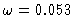, t is a normalized time, t=1 corresponds to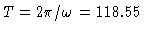.

Figure 6plane for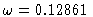, asymmetric oscillation.

Figure 7
Bifurcation diagram of a closed branch of asymmetric solutions, u(0) versus.

Figure 8
Same as in Figure 7, butversus.

Figure 9
Duffing equation,plane, Poincaré set of the stroboscopic map,, fractal dimension D=2.381.

This Example
Figure 1
Figure 2
Figure 3
Figure 4
Figure 5
Figure 6
Figure 7
Figure 8
Figure 9